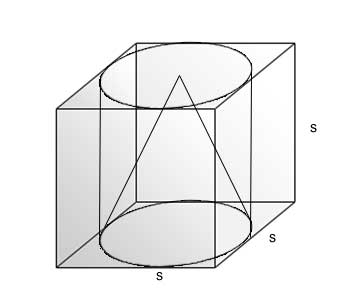SEARCH HOMEMath Central Quandaries & Queriesrohan, a student: A CYLINDER IS WITHIN THE CUBE TOUCHING ALL THE VERTICALS FACES . A CONE IS INSIDE THE CYLINDER.IF THEIR HEIGHT ARE SAME WITH SAME BASE ,FIND THE RATIO OF THEIR VOLUMES.Hi Rohan,

Consider what the objects look like together:Since the bases of the cylinder and the cone both have diameters of s (the length of one side of the cube), each of their radii is s/2. Both also have a height of s.

The formula for volume of a cylinder is

V = πr2h

The formula for volume of a cone is

V = πr2h/3

If you substitute in the radius and height for both, what is the ratio of their volumes?

Hope this helps,

JaniceMath Central is supported by the University of Regina and The Pacific Institute for the Mathematical Sciences.